Short articles on topics in systematic trading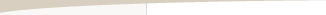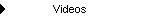Other Articles...

Performance statisticsPerformance Statistics

by Michael R. Bryant

Evaluating a trading system or method by testing it on historical data is essentially the same as simulating how that system will perform when it's traded. It follows, then, that the more accurate the simulation, the better the system can be evaluated. An important aspect of trading is the number of contracts or shares to take for each trade; i.e., position sizing. Including position sizing in a trading simulation makes it much easier to determine (1) the minimum account size needed to trade the system or method, (2) the initial position size for a given account size, (3) the expected return on account equity, and (4) the likely risk or return/risk ratio.

While including position sizing in a trading simulation can enhance the simulation, the performance summaries available in most trading software packages, such as TradeStation,™ are generally based on fixed contract trading; e.g., one contract per trade. It's usually possible to change the number of contracts for each trade (for example, using EasyLanguage programming in TradeStation); however, performance statistics such as the average trade, largest loss, and drawdown will still be quoted in dollars. This can make evaluating a trading system difficult. Why is this?

In most position sizing methods applicable to stock or futures trading, the number of contracts or shares tends to increase over time as trading profits accrue. As the number of contracts increases, so does the drawdown. A drawdown of 10% is \$3,000 on a \$30,000 account, but if the account grows to \$200,000, a 10% drawdown is \$20,000. If you are given the dollar value of the drawdown without the corresponding percentage, how do you know whether that \$20,000 drawdown is a 67% drawdown on the starting equity of \$30,000 or a 10% drawdown on the same account after it grew to \$200,000? The point is that the more important statistic is the percentage drawdown, not the absolute dollar value.

The same is true of the average trade size. It is more meaningful to know that the average trade size is 2% of equity than \$200. That \$200 average trade might be the average of trades with between zero and 10 contracts, for example. If you plan to start trading with a \$20,000 account, what size average trade should you expect? If you knew the average trade was 2% of equity, you could simply multiply your starting equity by 0.02 to find that the average trade will be \$400 initially.

The solution is to track trading results relative to account equity. That way, regardless of whether you're looking at one-contract results or fixed ratio position sizing, the performance statistics will be meaningful and the account size and position sizing will be properly represented.

Consider, for example, the performance results shown below. Notice that, where applicable, the statistic is calculated relative to account equity in percentage terms. The same statistic quoted in dollars can also be provided for comparison. Some statistics, such as the Modified Sharpe Ratio and the Return/Drawdown Ratio, use percentages exclusively.

Initial Account Equity: \$50000.00
Commissions and Slippage: \$0.00
Position Sizing Method: Fixed Percent Risk
Fixed Fraction: 5.00%

PROFIT SUMMARY
Total Net Profit: \$217099.03
Gross Profit: \$416111.21
Gross Loss: -\$199012.18
Profit Factor: 2.0909
Final Account Equity: \$267099.03
Return on Starting Equity: 434.20%

Percent Profitable: 54.00%
Max Number of Contracts: 49
Minimum Number of Contracts: 5
Average Number of Contracts: 15
Largest Winning Trade (\$): \$68566.36 (30.27%)
Largest Winnning Trade (%): 30.41% (\$18718.83)
Max Number Consecutive Wins: 7
Largest Losing Trade (\$): -\$30395.46 (-29.96%)
Largest Losing Trade (%): -30.20% (-\$16616.23)
Max Number Consecutive Losses: 6
Std Dev of Ave Trade (\$): \$11012.66
Std Dev of Ave Trade (%): 10.19%
Win/Loss Ratio (\$/\$): 1.7811
Win/Loss Ratio (%/%): 1.7044
Return/Drawdown Ratio: 11.9319
Modified Sharpe Ratio: 0.2163

DRAWDOWNS
Number of Closed Trade Drawdowns: 15
Average Drawdown (\$): -\$10416.93
Average Drawdown (%): 10.46%
Average Number of Trades in Drawdowns: 4
Worst Case Drawdown (\$): -\$30878.99 (30.29%)
Number of Trades in Drawdown: 11
Worst Case Drawdown (%): 36.39% (-\$30571.98)
Number of Trades in Drawdown: 22

Some of these statistics depend on the order of the trades; for example, the number of consecutive losses and the drawdown statistics. Except when one-contract position sizing is used, many others will also depend on the order of the trades. One way to account for trade ordering effects is with Monte Carlo analysis. If the statistics are compiled for each sample of the Monte Carlo simulation, they can be stated in terms of the Monte Carlo simulation results. The effect is that a confidence level will be added to each statistic, which will make the results even more useful.

The equity curve for a series of trades can be simulated more realistically when position sizing is included in the trading simulation. Using performance statistics based on account equity and expressed in percentage terms, such as many of the statistics shown above, makes it possible to better evaluate the equity curve and the corresponding trading system or method.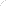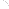Join Our Email List Email: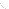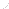For Email Marketing you can trust